# RD Sharma Class 8 Solutions Chapter 13 Profits, Loss, Discount and Value Added Tax (VAT) Ex 13.2

In this chapter, we provide RD Sharma Class 8 Solutions Chapter 13 Profits, Loss, Discount and Value Added Tax (VAT) Ex 13.2 for English medium students, Which will very helpful for every student in their exams. Students can download the latest RD Sharma Class 8 Solutions Chapter 13 Profits, Loss, Discount and Value Added Tax (VAT) Ex 13.2 Maths pdf, free RD Sharma Class 8 Solutions Chapter 13 Profits, Loss, Discount and Value Added Tax (VAT) Ex 13.2 book pdf download. Now you will get step by step solution to each question.

### RD Sharma Solutions for Class 8 Chapter 13 Profits, Loss, Discount and Value Added Tax (VAT) Ex 13.2Download PDF

Question 1.
Find the S.P. If
(i) M.P. = Rs. 1300 and Discount = 10%
(ii) M.P. = Rs. 500 and Discount = 15%
Solution:
(i) M.P. = Rs. 1300, Discount = 10%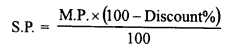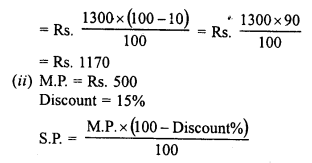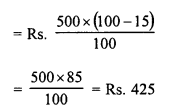Question 2.
Find the M.P. If
(i) S.P. = Rs. 1222 and Discount = 6%
(ii) S.P. = Rs. 495 and Discount = 1%
Solution:
(i) S.P. = Rs. 1222, discount = 6%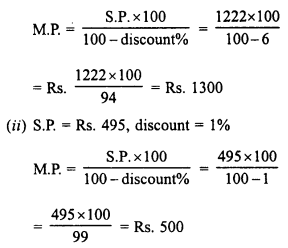Question 3.
Find discount in percent when
(i) M.P. = Rs. 900 and S.P. = Rs. 873
(ii) M.P. = Rs. 500 and S.P. = Rs. 425
Solution:
(i) M.P. = Rs. 900
S.P. = Rs. 873
Discount = M.P. – S.P. = Rs. 900 – Rs. 873 = Rs. 27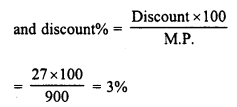(ii) M.P. = Rs. 500
S.P. = Rs. 425
Discount M.P. – S.P. = Rs. 500 – Rs. 425 = = Rs. 75Question 4.
A shop selling sewing machines offers 3% discount on ail cash purchases. What cash amount does a customer pay for a sewing machine, the price of which is marked as Rs. 650.
Solution:
Marked price (M.P.) of one sewing machine = Rs. 650
Rate of discount = 3%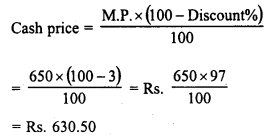Question 5.
The marked price of a ceiling fan is Rs. 720. During off season, it is sold for Rs. 684. Determine the discount percent.
Solution:
Marked price (M.P.) of fan = Rs. 720
Sale price (S.P.) = Rs. 684
Amount of discount = M.P. – S.P. = Rs. 720 – Rs. 684 = Rs. 36Question 6.
On the eve of Gandhi Jayanti, a saree is sold for Rs. 720 after allowing 20% discount. What is the marked price ?
Solution:
S.P. of saree = Rs. 720
Rate of discount = 20%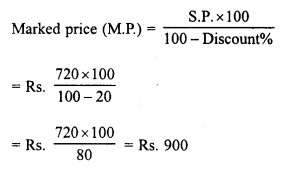Question 7.
After allowing a discount of 712 % on the marked price, an article is sold for Rs. 555. Find its marked price.
Solution:
Selling price (S.P.) = Rs. 555Question 8.
A shopkeeper allows his customers 10% off on the marked price of goods and still gets a profit of 25%. What is the actual cost to him of an article marked Rs. 250?
Solution:
Marked price = Rs. 250
Discount allowed = 10%Question 9.
A shopkeeper allows 20% off on the marked price of goods and still gets a profit of 25%. What is the actual cost to him of an article marked Rs. 500 ?
Solution:
Marked price (M.P.) of an article = Rs. 500
Discount allowed = 20%
Selling price (S.P.)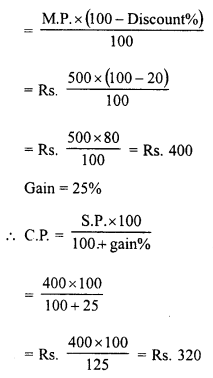Question 10.
A tradesman marks his goods at such a price that after allowing a discount of 15%, he makes a profit of 20%. What is the marked price of an article whose cost price is Rs 170 ?
Solution:
Rate of discount = 15% gain = 20%
Cost price (C.P.) of an article = Rs 170
Selling price (S.P.)Question 11.
A shopkeeper marks his goods in such a way that after allowing a discount of 25% on the marked price, he still makes a profit of 50%. Find the ratio of the C.P. to the M.P.
Solution:
Let cost price (C.P.) = Rs 100
Profit = 50%
Selling Price (S.P.)Question 12.
A cycle dealer offers a discount of 10% and still makes a profit of 26%. What is the actual cost to him of a cycle whose marked price is Rs 840 ?
Solution:
Rate of discount = 10%
Gain = 26%
Marked Price (M.P.) = Rs 840
Selling Price (S.P.)Question 13.
A shopkeeper allows 23% commission on his advertised price and still makes a profit of 10%. If he gains Rs 56 on one item, find his advertised price.
Solution:
Rate of commission = 23%
Profit = 10%
Total gain = Rs 56Question 14.
A shopkeeper marks his goods at 40% above the cost price but allows a discount of 5% for cash payment to his customers. What actual profit does he make, if he receives Rs 1064 after paying the discount ?
Solution:
Let cost price (C.P.) = Rs 100
Marked price = Rs 100 + 40 = Rs 140
Rate of discount = 5%
Selling price (S.P)Question 15.
By selling a pair of ear rings at a discount of 25% on the marked price, a jeweller makes a profit of 16%. If the profit is Rs 48, what is the cost price ? What is the marked price and the price at which the pair was eventually bought ?
Solution:
Total profit = Rs 48
Profit percent = 16%
Cost price = 48×10016 = Rs 300
Selling Price = C.P. + profit = Rs 300 + Rs 48 = Rs 348
Rate of discount = 25%Question 16.
A publisher gives 32% discount on the printed price of a book to booksellers. What does a bookseller pay for a book whose printed price is Rs 275 ?
Solution:
Printed price of a book = Rs 275
Rate of discount = 32%
Selling price (S.P.)Question 17.
After allowing a discount of 20% on the marked price of a lamp, a trader loses 10%. By what percentage is the marked price above the cost price ?
Solution:
Rate of discount = 20%
Loss = 10%
Let the cost price of the lamp = Rs 100
Loss = 10%
Selling price = Rs 100 – 10 = Rs 90
Rate of discount = 20%Question 18.
The list price of a table fan is Rs 480 and it is available to a retailer at 25% discount. For how much should a retailer sell it to gain 15% ?
Solution:
List price of table fan (M.P.) = Rs 480
Rate of discount = 25%
Selling price (S.P)Question 19.
Rohit buys an item at 25% discount on the marked price. He sells it for Rs 660, making a profit of 10%. What is the marked price of the item ?
Solution:
Rate of discount = 25%
Selling price (S.P.) for Rohit = Rs 660
Profit = 10%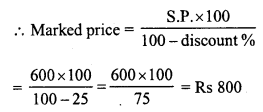Question 20.
A cycle merchant allows 20% discount on the marked price of the cycles and still makes a profit of 20%. If he gains Rs 360 over the sale of one cycle, find the marked price of the cycle.
Solution:
Rate of discount = 20%
Profit = 20%
Total gain = Rs 360Question 21.
Jyoti and Meena run a ready-made garment shop. They mark the garments at such a price that even after allowing a discount of 12.5%, they make a profit of 10%. Find the marked price of a suit which costs them Rs 1470.
Solution:
Rate of discount = 12.5%
Profit = 10%
Cost price = Rs 1470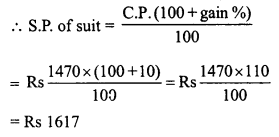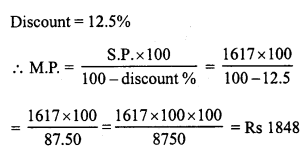Question 22.
What price should Aslam mark on a pair of shoes ? Which costs him Rs 1200 so as to gain 12% after allowing a discount of 16% ?
Solution:
Cost price of shoes = Rs 1200
Gain = 12%Question 23.
Jasmine allows 4% discount on the marked price of her goods and still earns a profit of 20%. What is the cost price of a shirt for her marked at Rs 850 ?
Solution:
Marked price of a shirt = Rs 850
Discount = 4%
Selling price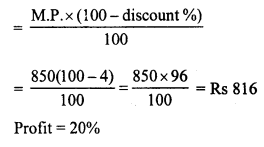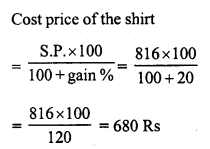Question 24.
A shopkeeper offers 10% off-season discount to the customers and still makes a profit of 26%. What is the cost price for the shopkeeper on a pair of shoes marked at Rs 1120 ?
Solution:
Marked price = Rs 1120
Rate of discount = 10%
S.P. of shoesQuestion 25.
A lady shopkeeper allows her customers 10% discount on the marked price of the goods and still gets a profit of 25%. What is the cost price of a fan for her marked at Rs 1250 ?
Solution:
Marked price (M.P.) of fan = Rs 1250
Discount = 10%
S.P. of the fan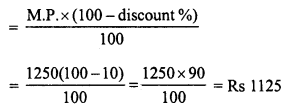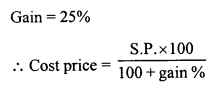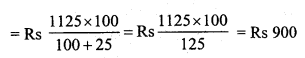All Chapter RD Sharma Solutions For Class 8 Maths

—————————————————————————–

All Subject NCERT Exemplar Problems Solutions For Class 8

All Subject NCERT Solutions For Class 8

*************************************************

I think you got complete solutions for this chapter. If You have any queries regarding this chapter, please comment on the below section our subject teacher will answer you. We tried our best to give complete solutions so you got good marks in your exam.

If these solutions have helped you, you can also share rdsharmasolutions.in to your friends.